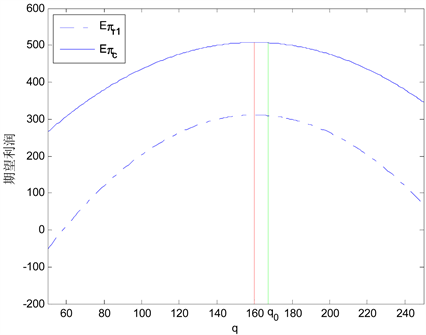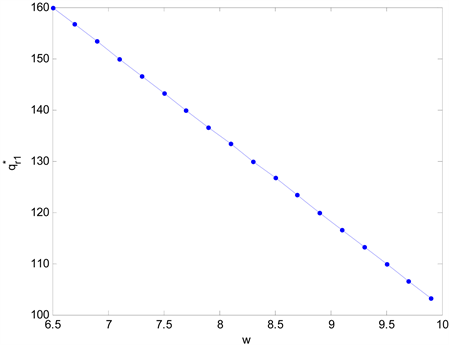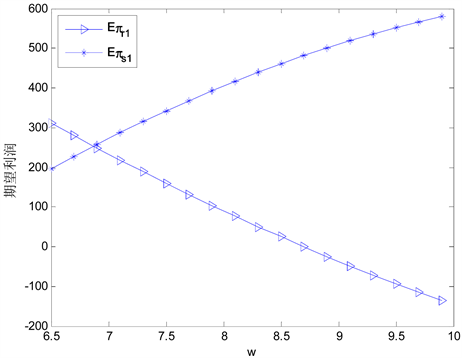# 需求依赖库存水平且零售商为库存风险厌恶的供应链协调Supply Chain Coordination with Inventory Level Dependent Demand and Inventory Risk Averse Retailer

• 全文下载: PDF(1298KB)    PP.80-92   DOI: 10.12677/ORF.2019.91010
• 下载量: 319  浏览量: 436   国家自然科学基金支持

The coordination issue of supply chain composed of a supplier and a retailer is discussed, in which the demand of the product is stochastic and affected by the inventory level, that is, when the in-ventory level is high, the demand increases, and when the inventory level is low, the demand de-creases. Taking the probability of surplus products exceeding the predetermined valve as the re-tailer’s inventory risk measurement, the retailer’s ordering strategy is analyzed and the way to achieve supply chain coordination by the rebate and penalty contract is explored. The results show that the rebate and penalty contract can coordinate the supply chain with certain conditions. Numerical analysis shows that with the increase of demand elasticity parameter, the optimal order quantity and the supply chain overall profit increase. However, with the increase of inventory risk averse, the optimal order quantity may decrease, then the supply chain overall profit decreases and the supply chain fails to coordinate.

1. 引言

2. 问题描述

$D\left(q,x\right)=x+\alpha \left(q-x-u\right)$(1)

$\mathrm{Pr}\left\{q-s\left(q,x\right)\ge v\right\}\le \xi$(2)

$\begin{array}{c}s\left(q,x\right)=\mathrm{min}\left(D\left(q,x\right),q\right)\\ =\left\{\begin{array}{ll}x+\alpha \left(q-x-u\right),\hfill & 0\le x\le q+\theta u;\hfill \\ q,\hfill & x>q+\theta u,\hfill \end{array}\end{array}$ (3)

$\begin{array}{c}S\left(q\right)={\int }_{0}^{q+\theta u}\left[x+\alpha \left(q-x-u\right)\right]f\left(x\right)\text{d}x+{\int }_{q+\theta u}^{+\infty }qf\left(x\right)\text{d}x\\ =q-\left(1-\alpha \right){\int }_{0}^{q+\theta u}F\left(x\right)\text{d}x.\end{array}$

$\begin{array}{c}I\left(q\right)=E{\left(q-s\left(x,q\right)\right)}^{+}\\ \text{=}{\int }_{0}^{q+\theta u}\left[q-x-\alpha \left(q-x-u\right)\right]f\left(x\right)\text{d}x\\ \text{=}\left(\text{1}-\alpha \right){\int }_{0}^{q+\theta u}F\left(x\right)\text{\hspace{0.17em}}\text{d}x.\end{array}$

${q}_{0}={F}^{-1}\left(\xi \right)-\left(\alpha u-v\right)/\left(1-\alpha \right)$(4)

$\begin{array}{c}\mathrm{Pr}\left\{q-s\left(q,x\right)\ge v\right\}=\mathrm{Pr}\left\{q-s\left(q,x\right)\ge v,x\le q+\theta u\right\}\\ +\mathrm{Pr}\left\{q-s\left(q,x\right)\ge v,x>q+\theta u\right\}\\ =\mathrm{Pr}\left\{x\le q+\left(\alpha u-v\right)/\left(1-\alpha \right)\right\}\\ =F\left(q+\left(\alpha u-v\right)/\left(1-\alpha \right)\right).\end{array}$

3. 集中式供应链

$\begin{array}{c}E{\text{π}}_{c}\left(q\right)=pS\left(q\right)+hI\left(q\right)-cq\\ =\left(p-c\right)q-\left(1-\alpha \right)\left(p-h\right){\int }_{0}^{q+\theta u}F\left(x\right)\text{d}x.\end{array}$ (5)

$\left(1-\alpha \right)F\left(\theta u\right)\le \frac{p-c}{p-h}<1-\alpha$(6)

${q}_{c}={F}^{-1}\left(\frac{p-c}{\left(1-\alpha \right)\left(p-h\right)}\right)-\theta u$(7)

$\frac{\text{d}E{\text{π}}_{c}\left(q\right)}{\text{d}q}=\left(p-c\right)-\left(1-\alpha \right)\left(p-h\right)F\left(q+\theta u\right)$

$\frac{{\text{d}}^{2}E{\pi }_{c}\left(q\right)}{\text{d}{q}^{2}}=-\left(1-\alpha \right)\left(p-h\right)f\left(q+\theta u\right)$

$\underset{q\to 0}{\mathrm{lim}}\frac{\text{d}E{\text{π}}_{c}\left(q\right)}{\text{d}q}=\left(p-c\right)-\left(1-\alpha \right)\left(p-h\right)F\left(\theta u\right)\ge 0$

$\underset{q\to \infty }{\mathrm{lim}}\frac{\text{d}E{\text{π}}_{c}\left(q\right)}{\text{d}q}=\left(p-c\right)-\left(1-\alpha \right)\left(p-h\right)<0$

$\alpha =0$ 时，供应链系统的最优订购量为：

${q}_{c}={F}^{-1}\left(\frac{p-c}{p-h}\right)$

4. 分散式供应链

4.1. 批发价契约

$\begin{array}{c}E{\text{π}}_{r}\left(q\right)=pS\left(q\right)+hI\left(q\right)-wq\\ =\left(p-w\right)q-\left(1-\alpha \right)\left(p-h\right){\int }_{0}^{q+\theta u}F\left(x\right)\text{d}x.\end{array}$ (8)

$E{\text{π}}_{s}\left(q\right)=\left(w-c\right)q$(9)

$\begin{array}{ll}\mathrm{max}\hfill & E{\text{π}}_{r}\left(q\right)\hfill \\ s.t.\hfill & 0 (10)

$\left(1-\alpha \right)F\left(\theta u\right)\le \frac{p-w}{p-h}<1-\alpha$(11)

${q}_{r}={F}^{-1}\left(\frac{p-w}{\left(1-\alpha \right)\left(p-h\right)}\right)-\theta u$(12)

$\frac{\text{d}E{\text{π}}_{r}\left(q\right)}{\text{d}q}=\left(p-w\right)-\left(1-\alpha \right)\left(p-h\right)F\left(q+\theta u\right)$

$\frac{{\text{d}}^{2}E{\text{π}}_{r}\left(q\right)}{\text{d}{q}^{2}}=-\left(1-\alpha \right)\left(p-h\right)f\left(q+\theta u\right)$

$\underset{q\to 0}{\mathrm{lim}}\frac{\text{d}E{\text{π}}_{r}\left(q\right)}{\text{d}q}=\left(p-w\right)-\left(1-\alpha \right)\left(p-h\right)F\left(\theta u\right)\ge 0$

$\underset{q\to \infty }{\mathrm{lim}}\frac{\text{d}E{\text{π}}_{r}\left(q\right)}{\text{d}q}=\left(p-w\right)-\left(1-\alpha \right)\left(p-h\right)<0$

4.2. 回馈与惩罚契约

$\begin{array}{c}E{\text{π}}_{r1}\left(q\right)=pS\left(q\right)+hI\left(q\right)+\beta E\left(s\left(q\right)-T\right)-wq\\ \text{=}\left(p-w+\beta \right)q-\left(1-\alpha \right)\left(p-h+\beta \right){\int }_{0}^{q+\theta u}F\left(x\right)\text{d}x-\beta T.\end{array}$ (13)

$\begin{array}{c}E{\text{π}}_{s1}\left(q\right)=\left(w-c\right)q-\beta E\left(s\left(q\right)-T\right)\\ \text{=}\left(w-c-\beta \right)q+\beta \left(1-\alpha \right){\int }_{0}^{q+\theta u}F\left(x\right)\text{d}x+\beta T.\end{array}$ (14)

$\begin{array}{ll}\mathrm{max}\hfill & E{\text{π}}_{r1}\left(q\right)\hfill \\ s.t.\hfill & 0 (15)

$\left(1-\alpha \right)F\left(\theta u\right)\le \frac{p-w+\beta }{p-h+\beta }<1-\alpha$(16)

${q}_{r1}={F}^{-1}\left(\frac{p-w+\beta }{\left(1-\alpha \right)\left(p-h+\beta \right)}\right)-\theta u$(17)

$\frac{\text{d}E{\text{π}}_{r1}\left(q\right)}{\text{d}q}=p-w+\beta -\left(1-\alpha \right)\left(p-h+\beta \right)F\left(q+\theta u\right)$

$\frac{{\text{d}}^{2}E{\text{π}}_{r1}\left(q\right)}{\text{d}{q}^{2}}=-\left(p-h+\beta \right)f\left(q+\theta u\right)$

$\underset{q\to 0}{\mathrm{lim}}\frac{\text{d}E{\text{π}}_{r1}\left(q\right)}{\text{d}q}=p-w+\beta -\left(1-\alpha \right)\left(p-h+\beta \right)F\left(\theta u\right)\ge 0$

$\underset{q\to \infty }{\mathrm{lim}}\frac{\text{d}E{\text{π}}_{r1}\left(q\right)}{\text{d}q}=p-w+\beta -\left(1-\alpha \right)\left(p-h+\beta \right)<0$

$E{\text{π}}_{r1}^{*}=E{\text{π}}_{r1}\left({q}_{c}\right)$$E{\text{π}}_{s1}^{*}=E{\text{π}}_{s1}\left({q}_{c}\right)$$E{\text{π}}_{c}^{*}=E{\text{π}}_{c}\left({q}_{c}\right)$$E{\text{π}}_{r}^{*}=E{\text{π}}_{r}\left({q}_{r}\right)$$E{\text{π}}_{s}^{*}=E{\text{π}}_{s}\left({q}_{r}\right)$。接下来，我们探讨用回馈与惩罚契约来实现供应链协调的途径。

$\left(1-\alpha \right)F\left(\theta u\right)\le \frac{p-c}{p-h}\le \left(1-\alpha \right)F\left({F}^{-1}\left(\zeta \right)+\frac{v}{1-\alpha }\right)$(18)

$\beta =\frac{p-h}{c-h}w-\frac{p-h}{c-h}c$(19)

${T}_{1}\le T\le {T}_{2}$(20)

${T}_{1}=\frac{\left(c-h\right)E{\text{π}}_{s}^{*}+\left(w-c\right)E{\text{π}}_{c}^{*}}{\left(p-h\right)\left(w-c\right)}$

${T}_{2}=\frac{\left(w-h\right)E{\text{π}}_{c}^{*}-\left(c-h\right)E{\text{π}}_{r}^{*}}{\left(p-h\right)\left(w-c\right)}$

$\frac{p-w+\beta }{p-h+\beta }=\frac{p-c}{p-h}$(21)

$\left(1-\alpha \right)F\left(u\theta \right)\le \frac{p-c}{p-h}\le \left(1-\alpha \right)F\left({F}^{-1}\left(\zeta \right)+\frac{v}{1-\alpha }\right)<1-\alpha$(22)

$\left\{\begin{array}{c}E{\text{π}}_{r1}^{*}\ge E{\text{π}}_{r}^{*}\\ E{\text{π}}_{s1}^{*}\ge E{\text{π}}_{s}^{*}\end{array}$

$E{\text{π}}_{r1}^{*}=\frac{w-h}{c-h}E{\text{π}}_{c}^{*}-\frac{\left(p-h\right)\left(w-c\right)}{c-h}T$(23)

$E{\text{π}}_{s1}^{*}=\frac{c-w}{c-h}E{\text{π}}_{c}^{*}+\frac{\left(p-h\right)\left(w-c\right)}{c-h}T$(24)

${T}_{1}\le T\le {T}_{2}$ 时， $E{\text{π}}_{s1}^{*}\ge E{\text{π}}_{s}^{*}$$E{\text{π}}_{r1}^{*}\ge E{\text{π}}_{r}^{*}$。此时，供应链的协调定义的三个条件均得到满足，则供应链实现了协调。

5. 数值例子

5.1. 协调分析Figure 1. The expected profit curves of the retailer and the supply chain systemTable 1. The impacts of T on E π r 1 * and E π s 1 *Table 2. The impacts of α on q r 1 * and T

5.2. 参数对供应链的影响

5.2.1. 参数w和βFigure 2. The impacts of w on the optimal order quantityFigure 3. The impacts of $w$ on $E{\text{π}}_{r1}$ and $E{\text{π}}_{s1}$

$\xi =0.6$$v=50$$w=6.5$$T=90$。将 $\beta$ 从0到6取值，绘制出最优订货量和供应链成员利润关于 $\beta$ 的曲线，如图4图5所示。Figure 4. The impacts of β on the optimal order quantityFigure 5. The impacts of $\beta$ on $E{\text{π}}_{r1}$ and $E{\text{π}}_{s1}$

5.2.2. 参数α

$w=6.5$$\beta =5$$u=60$$v=50$$\xi =0.6$$T=90$。当 $\alpha$ 变化时，结果如表3所示。Table 3. The impacts of α on the optimal order quantity and the supply chain profit

5.2.3. 参数ξ

$w=6.5$$\beta =5$$\alpha =0.2$$u=60$$T=90$，则 ${q}_{r1}={q}_{c}=160$。当 $\xi$ 变化时，结果如表4所示。Table 4. The impacts of ξ on the optimal order quantity and the supply chain profit

6. 结语

NOTES

*通讯作者。

  Gan, X., Sethi, S.P. and Yan, H. (2010) Channel Coordination with a Risk-Neutral Supplier and a Down-side-Risk-Averse Retailer. Production & Operations Management, 14, 80-89. https://doi.org/10.1111/j.1937-5956.2005.tb00011.x  Zhang, L., Song, S.J. and Wu, C. (2012) Supply Chain Coordination of Loss-Averse Newsvendor with Contract. Tsinghua Science & Technology, 10, 2-9.  Shi, K. and Xiao, T. (2008) Coordination of a Supply Chain with a Loss-Averse Retailer under Two Types of Contracts. In-ternational Journal of Information and Decision Sciences, 1, 5-25. https://doi.org/10.1504/IJIDS.2008.020033  Hu, B., Meng, C., Xu, D., et al. (2016) Three-Echelon Sup-ply Chain Coordination with a Loss-Averse Retailer and Revenue Sharing Contracts. International Journal of Production Economics, 179, 192-202. https://doi.org/10.1016/j.ijpe.2016.06.001  Hsieh, C.C. and Lu, Y.T. (2010) Manufacturer’s Return Policy in A Two-Stage Supply Chain with Two Risk-Averse Retailers and Random Demand. European Journal of Operational Research, 207, 514-523. https://doi.org/10.1016/j.ejor.2010.04.026  Chiu, C.H., Choi, T.M. and Li, X. (2011) Supply Chain Co-ordination with Risk Sensitive Retailer under Target Sales Rebates. Automatica, 47, 1617-1625. https://doi.org/10.1016/j.automatica.2011.04.012  Xu, G., Dan, B., Zhang, X., et al. (2014) Coordinating a Dual-Channel Supply Chain with Risk-Averse under a Two-Way Revenue Sharing Contract. International Journal of Production Economics, 147, 171-179. https://doi.org/10.1016/j.ijpe.2013.09.012  李建斌. 余牛. 刘志学. 两种基于CVaR准则的供应链返利与惩罚契约研究[J]. 系统工程理论与实践, 2015, 35(7): 1666-1677.  霍良安. 蒋杰辉. 赵玉苹. 等. 突发事件下回馈与惩罚契约协调的风险厌恶闭环供应链研究[J]. 计算机应用研究, 2017, 34(1): 31-35.  魏光兴. 邓欣. 杨程程. 零售商具有库存风险厌恶偏好的供应链部分回购契约[J]. 重庆交通大学学报(自然科学版), 2013, 32(5): 1082-1085.  Devangan, L., et al. (2013) Individually Rational Buyback Contracts with Inventory Level Dependent Demand. International Journal of Production Economics, 142, 381-387. https://doi.org/10.1016/j.ijpe.2012.12.014  Wang, K.H., Huang, Y.C. and Tung, C.T. (2016) A Re-turn-Policy Contract with a Stock-Dependent Demand and Inventory Shrinkages. Asia Pacific Management Review, 21, 154-160. https://doi.org/10.1016/j.apmrv.2016.02.001  Parthasarathi, G., Sarmah, S.P. and Jenamani, M. (2010) Impact of Price Sensitive and Stock Dependent Random Demand on Supply Chain Coordination. International Journal of Management Science & Engineering Management, 5, 72-80. https://doi.org/10.1080/17509653.2010.10671094  Parthasarathi, G., Sarmah, S.P. and Jenamani, M. (2011) Supply Chain Coordination under Retail Competition Using Stock Dependent Price-Setting Newsvendor Framework. Operational Research, 11, 259-279. https://doi.org/10.1007/s12351-010-0077-z